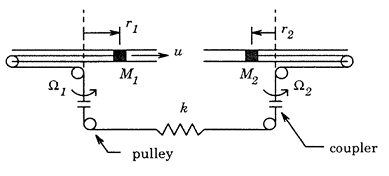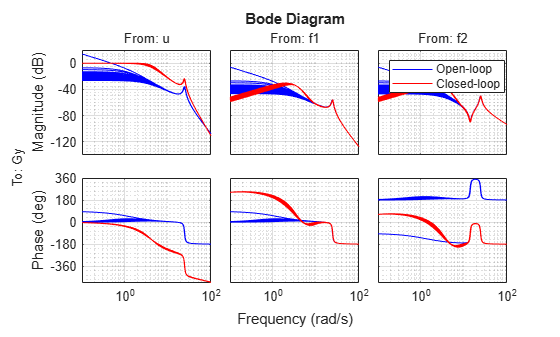# Control Design for Spinning Disks

This example shows how to design a gain-scheduled ${\mathit{H}}_{2}$ controller for a mechanical system consisting of coupled spinning disks described in .

### Coupled Spinning Disks

A pair of rotating disks is shown in this figure. The slotted disks rotate at rates of ${\Omega }_{1}$ rad/s and ${\Omega }_{2}$ rad/s. The disks contain masses ${\mathit{M}}_{1}$ and ${\mathit{M}}_{2}$, which can move in the horizontal plane and slide radially. The two masses are connected by a wire, coupler, and pulley system that can transmit force in both compression and tension. This coupling system acts as a spring with spring constant $\mathit{k}$, and friction due to the sliding motion of each mass is modeled by a damping coefficient $\mathit{b}$.The goal is to control the positions of the two masses: ${\mathit{r}}_{1}$ for mass ${\mathit{M}}_{1}$ and ${\mathit{r}}_{2}$ for ${\mathit{M}}_{2}$. The control input is a radial force ${\mathit{f}}_{\mathit{u}}$ acting on mass ${\mathit{M}}_{1}$ and radial disturbance forces ${\mathit{f}}_{1}$ and ${\mathit{f}}_{2}$ act on each mass. The equations of motions are as follows.

`${\mathit{M}}_{1}\left(\stackrel{¨}{\text{\hspace{0.17em}}{\mathit{r}}_{1}}-{\Omega }_{1}^{2}{\mathit{r}}_{1}\right)+\mathit{b}\stackrel{˙}{\text{\hspace{0.17em}}{\mathit{r}}_{1}}+\mathit{k}\left({\mathit{r}}_{1}+{\mathit{r}}_{2}\right)={\mathit{f}}_{\mathit{u}}+{\mathit{f}}_{1}$`

`${\mathit{M}}_{2}\left(\stackrel{¨}{\text{\hspace{0.17em}}{\mathit{r}}_{2}}-{\Omega }_{2}^{2}{\mathit{r}}_{2}\right)+\mathit{b}\stackrel{˙}{\text{\hspace{0.17em}}{\mathit{r}}_{2}}+\mathit{k}\left({\mathit{r}}_{1}+{\mathit{r}}_{2}\right)={\mathit{f}}_{2}$`

Here:

• ${\mathit{M}}_{1}$ = 1 kg and is the mass of the sliding mass in disk 1.

• ${\mathit{M}}_{1}$ = 2 kg and is the mass of the sliding mass in disk 2.

• $\mathit{b}$ = 1 kg/s and is the damping coefficient due to friction.

• $\mathit{k}$ = 200 N/m is the spring constant of mass coupling system.

• ${\mathit{r}}_{1}\left(\mathit{t}\right)$ is the position of the sliding mass ${\mathit{M}}_{1}$ relative to the center of disk 1 (m).

• ${\mathit{r}}_{2}\left(\mathit{t}\right)$ is the position of the sliding mass ${\mathit{M}}_{2}$ relative to the center of disk 2 (m).

• ${\Omega }_{1}\left(\mathit{t}\right)$ is the rotational rate of disk 1 (rad/s).

• ${\Omega }_{2}\left(\mathit{t}\right)$ is the rotational rate of disk 2 (rad/s).

• ${\mathit{f}}_{\mathit{u}}$ is the control force acting radially on mass ${\mathit{M}}_{1}$(N).

• ${\mathit{f}}_{1}$ and ${\mathit{f}}_{2}$ are the disturbance forces acting radially on ${\mathit{M}}_{1}$ and ${\mathit{M}}_{2}$, respectively (N).

The rotational rates of the spinning disks are allowed to vary in the ranges ${\Omega }_{1}\in \left[0,3\right]$ and ${\Omega }_{2}\in \left[0,5\right]$ (in rad/s). These rates are not known in advance but are measured and available for control design. The objective of the control design is to command the radial position of mass ${\mathit{M}}_{2}$. Note that the control input is applied to mass ${\mathit{M}}_{1}$ and is transmitted to mass ${\mathit{M}}_{2}$ through the disk coupling system.

### LPV Model

The equations of motions are linear except for the rates ${\Omega }_{1}$ and ${\Omega }_{2}$. Choosing ${\mathit{p}}_{1}={\Omega }_{1}^{2}$, ${\mathit{p}}_{2}={\Omega }_{2}^{2}$, ${\mathit{p}}_{1}\in \left[0,9\right]$, and ${\mathit{p}}_{2}\in \left[0,25\right]$ as scheduling parameters, you obtain the following LPV model of the overall system.

`$\stackrel{˙}{\mathit{x}}\left(\mathit{t}\right)=\left[\begin{array}{cccc}0& 0& 1& 0\\ 0& 0& 0& 1\\ {\mathit{p}}_{1}-\frac{\mathit{k}}{{\mathit{M}}_{1}}& -\frac{\mathit{k}}{{\mathit{M}}_{1}}& -\frac{\mathit{b}}{{\mathit{M}}_{1}}& 0\\ -\frac{\mathit{k}}{{\mathit{M}}_{2}}& {\mathit{p}}_{2}-\frac{\mathit{k}}{{\mathit{M}}_{2}}& 0& -\frac{\mathit{b}}{{\mathit{M}}_{2}}\end{array}\right]\mathit{x}\left(\mathit{t}\right)+\left[\begin{array}{ccc}0& 0& 0\\ 0& 0& 0\\ \frac{1}{{\mathit{M}}_{1}}& \frac{0.1}{{\mathit{M}}_{1}}& 0\\ 0& 0& \frac{0.1}{{\mathit{M}}_{2}}\end{array}\right]\left[\begin{array}{c}{\mathit{f}}_{\mathit{u}}\left(\mathit{t}\right)\\ {\mathit{f}}_{1}\left(\mathit{t}\right)\\ {\mathit{f}}_{2}\left(\mathit{t}\right)\end{array}\right]$`

`$\mathit{y}\left(\mathit{t}\right)=\left[\begin{array}{cccc}0& 1& 0& 0\end{array}\right]\mathit{x}\left(\mathit{t}\right)$`

Here, the state vector $\mathit{x}\left(\mathit{t}\right)=\left[\begin{array}{c}{\mathit{r}}_{1}\left(\mathit{t}\right)\\ {\mathit{r}}_{2}\left(\mathit{t}\right)\\ \stackrel{˙}{\text{\hspace{0.17em}}{\mathit{r}}_{1}}\left(\mathit{t}\right)\\ \stackrel{˙}{\text{\hspace{0.17em}}{\mathit{r}}_{2}}\left(\mathit{t}\right)\end{array}\right]$.

To create this LPV model, write a function `disksGFCN` that computes the $\mathit{A}$, $\mathit{B}$, $\mathit{C}$ matrices as a function of the scheduling parameters $\mathit{p}=\left[\begin{array}{c}{\mathit{p}}_{1}\\ {\mathit{p}}_{2}\end{array}\right]$. To see the code for this function, see the `disksGFCN.m` file or enter `type disksGFCN` at the command line.

Define system parameters.

```m1 = 1; m2 = 0.5; k = 200; b = 1;```

Define the LPV system.

```fh = @(t,p) disksGFCN(t,p,m1,m2,k,b); G = lpvss(["p1","p2"],fh);```

### Gain-Scheduled H2 Control Design

Design a gain-scheduled ${\mathit{H}}_{2}$-optimal controller. First, design an ${\mathit{H}}_{2}$-optimal controller at each point on a grid of (${{\Omega }_{1}^{2}}_{\text{\hspace{0.17em}}}$,${\Omega }_{2}^{2}$) values. For fixed rotational rates, such controllers minimize the closed-loop ${\mathit{H}}_{2}$ norm from disturbance $\mathit{d}=\left[\begin{array}{c}{\mathit{f}}_{1}\left(\mathit{t}\right)\\ {\mathit{f}}_{2}\left(\mathit{t}\right)\end{array}\right]$ to error $\mathit{e}$ for the weighted interconnection. For appropriately selected weights, this interconnection defines the desired ${\mathit{H}}_{2}$ performance of the control law. The controller takes in the reference $\mathit{r}$ and measurement $\mathit{y}$ and generates the command ${\mathit{u}}_{\mathit{c}}$.Define the weights and build an LPV model of this interconnection.

```% Add input/output names to plant G.InputName = {'u'; 'f1'; 'f2'}; G.OutputName = 'Gy'; % Actuator model act = ss(-100,100,1,0); act.InputName = 'uc'; act.OutputName = 'u'; % Weight: Control Command Wu = ss(1/50); Wu.InputName = 'uc'; Wu.OutputName = 'eu'; % Weight: Actuator Output Wa = ss(0.00001); Wa.InputName = 'u'; Wa.OutputName = 'ea'; % Weight: Tracking Error We = tf(2,[1 0.04]); We.InputName = 'e'; We.OutputName = 'er'; % Weight: Noise Wn = tf([1,0.4],[0.01 400]); Wn.InputName = 'dn'; Wn.OutputName = 'n'; % Form synthesis interconnection sum1 = sumblk('y = Gy + n'); sum2 = sumblk('e = Gy - r'); IC = connect(G,act,Wu,Wa,We,Wn,sum1,sum2,{'r','f1','f2','dn','uc'},... {'eu','ea','er','r','y'});```

Construct a grid of (${{\Omega }_{1}^{2}}_{\text{\hspace{0.17em}}}$,${\Omega }_{2}^{2}$) values and compute the ${\mathit{H}}_{2}$-optimal controller at each grid point.

```p1 = linspace(0,9,3); p2 = linspace(0,25,3); [p1,p2] = ndgrid(p1,p2); ```

Sample IC at grid points and design ${\mathit{H}}_{2}$-optimal controllers.

```nmeas = 2; % # of measurements ncon = 1; % # of controls ICs = sample(IC,[],p1,p2); Ks = ss( zeros([ncon nmeas size(p1)]) ); for i=1:numel(p1) Ks(:,:,i) = h2syn(ICs(:,:,i),nmeas,ncon); end```

Use `ssInterpolant` to create a gain-scheduled controller that linearly interpolates these ${\mathit{H}}_{2}$ controllers between grid points. This approach is valid when the rotational rates vary slowly. Alternative LPV methods for rapid changes are described in .

```Ks.SamplingGrid = struct('p1',p1,'p2',p2); Klpv = ssInterpolant(Ks,[]); Klpv.InputName = {'r','Gy'}; Klpv.OutputName = {'uc'};```

### LTI Performance Evaluation

Evaluate the gain-scheduled controller for fixed rotation rates using a finer grid than for the controller design.

Form closed-loop system from `[r;f1;f2]` to `Gy`.

`CL = connect(G,act,Klpv,{'r','f1','f2'},{'Gy'});`

Evaluate open and closed-loop on a finer grid of points.

```p1 = linspace(0,9,5); p2 = linspace(0,25,5); [p1,p2] = ndgrid(p1,p2); Gs = sample(G,[],p1,p2); CLs = sample(CL,[],p1,p2);```

The controller achieves good tracking with minimal overshoot, approximately 2.5 s settling time, and less than 3% steady-state tracking error. Furthermore, the disturbances have minimal effect.

```step(CLs,5) grid on```The closed-loop Bode response from `r` to `Gy` has a DC gain near one with a bandwidth near 3 rad/s. It shows good disturbance attenuation below 1 rad/s compared to the open loop.

```bode(Gs,"b",CLs,"r",{0.1,100}) legend("Open-loop","Closed-loop","Location","best") grid minor```### LPV Performance Evaluation

Finally, evaluate the gain-scheduled controller with a time-domain simulation with time-varying rotation rates. The parameters vary in time as follows:

${\mathit{p}}_{1}\left(\mathit{t}\right)={\Omega }_{1}^{2}\left(\mathit{t}\right)=\mathrm{sin}\left(\mathit{t}\right)+1.5$, ${\mathit{p}}_{2}\left(\mathit{t}\right)={\Omega }_{2}^{2}\left(\mathit{t}\right)=0.5\mathrm{cos}\left(5\mathit{t}\right)+3$.

The reference signal $\mathit{r}$ is a unit step and the disturbances are set to

${\mathit{f}}_{1}\left(\mathit{t}\right)=\mathrm{cos}\left(3\mathit{t}\right)+\mathrm{sin}\left(5\mathit{t}\right)+\mathrm{cos}\left(8\mathit{t}\right)$, ${\mathit{f}}_{2}\left(\mathit{t}\right)=\mathrm{cos}\left(\mathit{t}\right)+\mathrm{sin}\left(2\mathit{t}\right)+\mathrm{cos}\left(4\mathit{t}\right)$.

Define the inputs to the system.

```t = 0:0.01:15; u = ones(size(t)); d1 = cos(3*t)+sin(5*t)+cos(8*t); d2 = cos(t)+sin(2*t)+cos(4*t); ```

Define the trajectories of the parameters.

```p1 = sin(t)+1.5; p2 = 0.5*cos(5*t)+3; ```

Simulate the LPV model with and without disturbances.

```[y1,t1,x1] = lsim(CL,[u;d1;d2],t,[],[p1;p2]); [y2,t2,x2] = lsim(CL,[u;0*d1;0*d2],t,[],[p1;p2]); figure plot(t1,y1,"b",t2,y2,"r"); grid on; title("LPV Simulation") ylabel("y"); legend("With disturbances","Without disturbances","Location","best")```The error due to the disturbances is on the order of 5% and consists of 1–2 Hz oscillations about the disturbance-free response. This frequency range is incidentally where the LTI frequency response analysis indicates that disturbances have the greatest impact on the output signal.

### Data Function

`type disksGFCN.m`
```function [A,B,C,D,E,dx0,x0,u0,y0,Delays] = GFCN(t,p,m1,m2,k,b) % Matrices and offsets for LPV model of spinning disks. % Enforce 0<=p1<=9, 0<=p2<=25 p(1) = max(0,min(p(1),9)); p(2) = max(0,min(p(2),25)); % Define state-space matrices A = [ 0 0 1 0 ; ... 0 0 0 1 ; ... p(1)-k/m1 -k/m1 -b/m1 0 ; ... -k/m2 p(2)-k/m2 0 -b/m2 ]; B = [ 0 0 0 ; ... 0 0 0 ; ... 1/m1 .1/m1 0 ; ... 0 0 .1/m2 ]; C = [ 0 1 0 0]; D = [0 0 0]; E = []; % no offsets or delays dx0 = []; x0 = []; u0 = []; y0 = []; Delays = []; ```

### References

 Wu, Fen. "Control of Linear Parameter Varying Systems," Ph.D. dissertation, Department of Mechanical Engineering, University of California at Berkeley, May 1995.